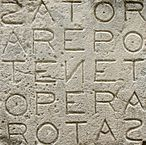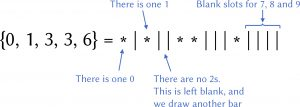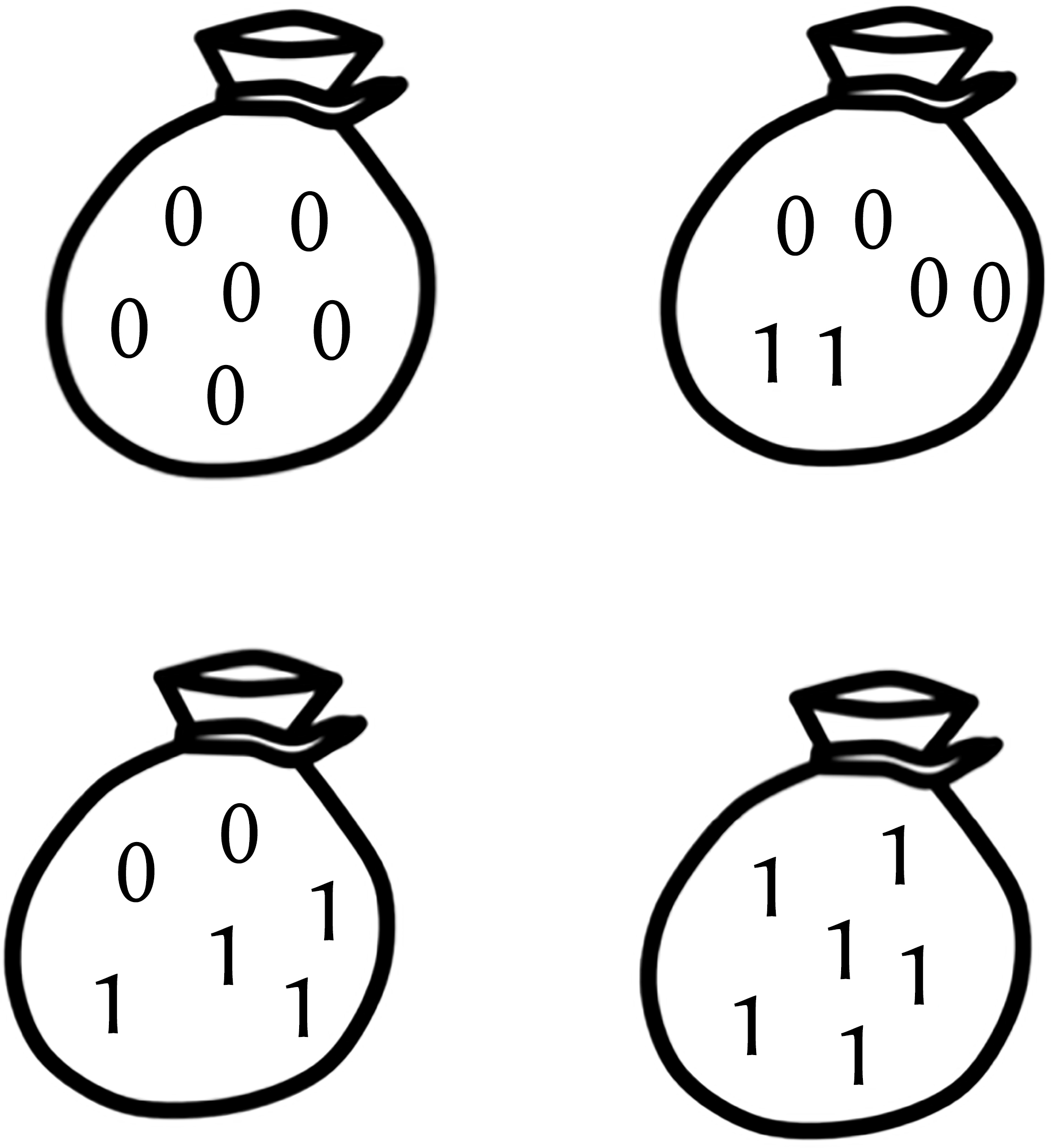# Counting palindromes

Alex Xela shows us the world of palindromic numbers, and calculates the chances of getting oneImage: Flickr user Chris, CC BY 2.0

About a year ago, I was walking to work when I started to think about palindromes. This was partly because I’d seen one at work the day before, but mostly because my iPod had packed up on me, leaving me with a good half an hour with nothing better to do.

A palindrome is a word like LEVEL, which reads the same forwards and backwards. Specifically, I was thinking about 5-digit numbers, so my palindromes were things like 01410. I’d seen one like this at work the previous day, which got me thinking-what were the chances of that happening? This turns out not to be a very interesting question to ask—for a random 5-digit number, the answer is simply 1 in 100. The first 3 digits can be whatever they like, and then the remaining two must match up with a 1-in-10 chance for each.The Sator Square (AD79), a word square containing a five-word Latin palindrome. M Disdero, CC BY-SA 3.0

I was still 25 minutes from work at this point, so I needed to make things harder. Next, I thought about what would happen if you threw re-arrangement into the mix. If a well-meaning person presented you with a bag of five numbers and wanted you to make a palindrome, what are the chances that you could do it?

To answer this, we need to first define exactly what is meant by one of these ‘bags’. In mathematical terms, I’m talking about unordered multisets. Unordered simply means that, for example, $\{1, 2, 3, 4, 5\}$ and $\{5, 4, 3, 2, 1\}$ are equal-they are considered to be two representations of the same set. And multiset means that the sets may contain repeated elements, so $\{1, 1, 2\}$ is a different set to $\{1, 2\}$. If it’s possible to make a palindrome from the individual elements, then I’ll call such a set palindromic.

To see how complicated this makes the problem, let’s think about something simpler for a moment. How many of these sets are there? When we were talking about 5-digit numbers, this was easy-there are 100,000 (it’s simply all the numbers from 00000 to 99999).

However, there are far fewer unordered sets of 5 digits. To see why this is true, consider the set $\{0, 3, 3, 8, 8\}$. This is a single set, but can produce 30 different 5-digit numbers (03388, 33088, 83830 to name a few). So, do we just divide by 30? Nope-the relationship isn’t even linear. For $\{1, 2, 3, 4, 5\}$ there are 120 rearrangements, and for $\{0, 0, 0, 0, 0\}$ there is only 1. Clearly this isn’t simple, although there is a known solution to this question which we’ll come to a bit later.

## Breaking the problem down

For the remainder of my commute I didn’t calculate the answer, but I did settle on an approach to complete when I returned home that evening. To solve the problem, I broke it down into cases. In fact, as I was thinking about 5 ‘things’ and how often they were repeated, I thought about it like a game of poker.

As an example, let’s think about the bags where all 5 digits are the same (“five-of-a-kind”). There are clearly 10 of these, and for any one of them you can make a palindrome. Similarly, there are 90 “four-of-a-kind” bags (of the form AAAAB: 10 choices for A, then 9 for B: $10 \times 9 = 90$) and 90 “full house” bags (of the form AAABB), all of which also produce palindromes.

Continuing in this way, the remaining cases are AAABC (“three of a kind”), AABBC (“two pair”), AABCD (“one pair”) and ABCDE (“high card”). Crunching the numbers reveals that 550 out of a total of 2002 bags are palindromic-the chance of finding a palindrome in the rephrased problem is now a little over 25%. Coincidentally, the total number of bags is itself a palindrome in this case! Well, that was a fun diversion—nothing more to see here, right?

Wrong. Now I wanted to solve the general problem, by which I mean two things. Firstly, let’s throw away the digits 0-9 and have an arbitrary alphabet of $b$ characters. That could be A-Z, it could be base 64—whatever we feel like.

And instead of bags of size 5, let’s make that arbitrary as well and call it $n$. Is there a general solution for this probability in terms of $b$ and $n$? It turns out that yes, there is, but it would be a couple of weeks before I found it.

## The general problem

The first step was to realise that counting unordered sets was something I’d encountered before, while studying combinatorics at university. I dug out my lecture notes and found the multinomial coefficient, which gives an expression in terms of $n$ and $b$ for how many unordered multisets there are in total:

$$\binom{n+b-1}{b-1} = \frac{(n+b-1)!}{(\hspace{-1pt}n\hspace{1pt})! \, (b-1)!}.$$

Plugging in $n = 5$ and $b = 10$ gives us 2002, which matches the number we manually worked out earlier on. To see why it’s true in general, we must first rephrase the problem-something which happens a lot in combinatorics proofs. Suppose that we choose to represent our sets in a different way. We’ll agree an order for our alphabet (eg A-Z, or 0-9), and use it to write out a particular set as follows. Starting with the first character in the alphabet, we’ll draw a star for any that are present in our set. Then, we’ll draw a single line to show that we’re onto the next character, and repeat the process. This is much easier to demonstrate with an example:With a little mathematical rigour, you can show that these two representations are equivalent, meaning we can instead choose to count how many ways we can draw out $n$ stars and $b-1$ lines. The total number of characters is $n+b-1$, and we must choose $b-1$ of them to be lines. This is our answer!

This was brilliant—most of my work was done for me. Now I just needed to work out how to count the palindromic ones for the general case.

## Two tricks

There were two ‘tricks’ that got me to the solution. The first was something I spotted almost straight away, but its significance didn’t become clear to me until later. There is a noticeable pattern in the number of palindromic sets as $n$ oscillates between odd and even. To see what I mean, here are the numbers where $b = 10$ (the digits 0-9, say) and we let $n$ increase:

 Bag size, n 2 3 4 5 6 7 8 9 Palindromic sets 10 100 55 550 220 2200 715 7150

The number of palindromic sets multiplies by 10 every time we increase from an even number to an odd number. More generally, it multiplies by $b$, and when you think about it the reason becomes clear. In an even-sized set, every element must be paired for a palindrome to be possible. When we increase the size by one, we can put any of our $b$ elements in there and it’ll still be palindromic-the new element can just go in the middle! Mathematically, if we label the set of palindromic sets over $n$ and $b$ as $P^{\, n}_b$ then the result is:

$$|P_b^{\, \, 2n \, + \, 1} | = b \, | P_b^{\, \, 2n}| \qquad \forall n,\, b.$$

This fact is interesting, but it feels like we still have the bulk of the work to do. All we’ve shown is that if we can solve one of the even or odd cases, then we’ve finished the problem. And that’s where the next trick came in, focusing on the even case.

Again, I’ll illustrate this with a simple example. This time I’m going to restrict us to an alphabet of $\{0, 1\}$ to make things more manageable:(a) All bags for $n=3$(b) All palindromic bags for $n=6$

What’s illustrated here is that the number of palindromic bags of even size is the same as the total number of bags that are half the size. Just take every bag from the space half the size and double up all the elements-everything you’ve just created is unique and palindromic, and you can show that every palindromic set can be created this way. And, crucially, we know the total number of bags in the smaller space because that’s the multinomial coefficient. We’ve done it!

The final form is as follows, where $M(n,b)$ is the multinomial coefficient for $n$ and $b$:

$$M(n,b) = \begin{cases} \cfrac{M(\frac{n}{2},b) }{M(n,b)},\text{ if }n\text{ is even,} \\\\ b \, \cfrac{M (\frac{n-1}{2},b) }{M(n,b)},\text{ if }n\text{ is odd.}\end{cases}$$

## Convergence

My final area of interest before putting this whole thing to bed was to explore the limits of my closed form in $n$ and $b$. I was able to graph the function (pictured on the next page), and then thought I might as well do some analysis too. As it turned out, I was in for one last surprise.

The limit as $b$ increases isn’t very interesting-if we think about it logically for a second it’s obvious what should happen. For a fixed size of bag, if we just keep increasing the number of characters in our alphabet then obviously the probability of being able to make a palindrome converges to 0 (and pretty quickly, I might add).

However, things weren’t so simple when looking at the $n$ case. I expected this to go to 0 as well—it feels like by making the bag larger you’re making it increasingly difficult to find palindromes. This intuition is correct, but only partially. The probability does decrease as the bag size increases (ignoring the fluctuation between odd/even, of course). However, it doesn’t decrease to 0-there is a positive limit! This was exciting for me as it was completely unexpected. It also explained why my attempted proofs that it converged to 0 hadn’t been going very well…Plots of the palindromic density function

The limit in $n$ comes out as follows-something that can be shown using L’Hôpital’s rule:

$$\cfrac{1}{2^{b-1}},\mbox{ if }n\mbox{ is even,} \qquad \cfrac{b}{2^{b-1}},\mbox{ if }n\mbox{ is odd.}$$

But why is this? Can you come up with a logical reason for why this should be the case?

## An alternate problem

To conclude, I’ll leave you with an alternative version of the same problem. At the time of writing, I haven’t delved into it myself yet, so there may be other interesting results to be found. The re-phrased problem is this:

### Challenge

Given an alphabet of $b$ characters, pick $n$ elements at random (allowing duplicates). What is the probability that you can make a palindrome with the resulting set of elements? There is a subtle difference here, which I’ll leave for you to figure out. Is there a general solution for this version? Does it have interesting limits? Enjoy!Alex studied maths at the University of Warwick, graduating in 2013. He is a
software developer for a clinical software company based in Leeds. He is a qualified day skipper—when he’s not working or solving puzzles, you can find him sailing the Caribbean!